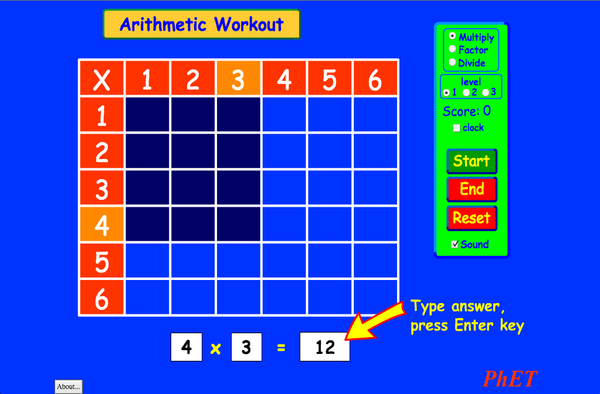# حسابدانلودEmbed کردن ببند نسخه فعلی این شبیه سازی را embed کنید از این فایل HTML برای embed کردن نسخه فعلی این شبیه سازی استفاده کنید. ضمنا میتوانید طول و عرض را عوض کنید. تصویری را embed کنید که با کلیک کردن روی آن شبیه سازی اجرا شود.
برای اجرا کلیک کنید
از این کد برای اجرای تصویری که پیغام میدهد "برای اجرا اینجا را کلیک کنید" استفاده کنید. ضرب PhET حمایت می شود توسطو مدرسانی چون شما.Back to HTML5 Version

• ضرب

### Description

شبیه سازی. حساب. توضیح
جدول ضربتان را به یاد می آورید؟..... من هم همینطور. خاطره جدول ضرب،تقسیم و مهارت های فاکتور گیری خود را با این بازی مهیج تکرارکنید. هیچ نوع ماشین حسابی مجاز نیست.

### نمونه ای از هدف های آموزشی

• شبیه سازی. حساب. اهداف یادگیری:
• توضیح دهد چگونه جداول ضرب به ضرب، فاکتور و تقسیم کمک می کنند
• افزایش دقت در ضرب کردن، فاکتور گیری و تقسیم.

### Standards Alignment

#### Common Core - Math

3.OA.A.1
Interpret products of whole numbers, e.g., interpret 5 × 7 as the total number of objects in 5 groups of 7 objects each. For example, describe a context in which a total number of objects can be expressed as 5 × 7.
3.OA.A.2
Interpret whole-number quotients of whole numbers, e.g., interpret 56 ÷ 8 as the number of objects in each share when 56 objects are partitioned equally into 8 shares, or as a number of shares when 56 objects are partitioned into equal shares of 8 objects each. For example, describe a context in which a number of shares or a number of groups can be expressed as 56 ÷ 8.
3.OA.A.4
Determine the unknown whole number in a multiplication or division equation relating three whole numbers. For example, determine the unknown number that makes the equation true in each of the equations 8 × ? = 48, 5 = _ ÷ 3, 6 × 6 = ?
3.OA.B.5
Apply properties of operations as strategies to multiply and divide.2 Examples: If 6 × 4 = 24 is known, then 4 × 6 = 24 is also known. (Commutative property of multiplication.) 3 × 5 × 2 can be found by 3 × 5 = 15, then 15 × 2 = 30, or by 5 × 2 = 10, then 3 × 10 = 30. (Associative property of multiplication.) Knowing that 8 × 5 = 40 and 8 × 2 = 16, one can find 8 × 7 as 8 × (5 + 2) = (8 × 5) + (8 × 2) = 40 + 16 = 56. (Distributive property.)
3.OA.B.6
Understand division as an unknown-factor problem. For example, find 32 ÷ 8 by finding the number that makes 32 when multiplied by 8.
3.OA.C.7
Fluently multiply and divide within 100, using strategies such as the relationship between multiplication and division (e.g., knowing that 8 × 5 = 40, one knows 40 ÷ 5 = 8) or properties of operations. By the end of Grade 3, know from memory all products of two one-digit numbers.
3.OA.D.9
Identify arithmetic patterns (including patterns in the addition table or multiplication table), and explain them using properties of operations. For example, observe that 4 times a number is always even, and explain why 4 times a number can be decomposed into two equal addends.
Version 2.03

### Teacher Tipsراهنمای معلم مجموعه توصیه های تولید شده توسط گروه PHET به صورت pdf. ( PDF ).

### Teacher-Submitted Activities

Arithmetic Games (Inquiry Based)Trish Loeblein دبیرستان آزمایشگاه ریاضیات
Juego de Aritmética (Basado en Indagación)Trish Loeblein, traducido por Diana López دبیرستان
راهنمایی
کار در خانه
Guided
ریاضیات
Alignment of PhET sims with NGSSTrish Loeblein دبیرستان سایر فیزیک
زیست شناسی
علوم زمین
شیمی
How do PhET simulations fit in my middle school program?Sarah Borenstein راهنمایی سایر زیست شناسی
شیمی
فیزیک
علوم زمین
MS and HS TEK to Sim Alignment Elyse Zimmer راهنمایی
دبیرستان
سایر زیست شناسی
فیزیک
شیمی
Math facts - Using the Multiplication Chart Tapan Sarkar راهنمایی آزمایشگاه ریاضیات
Back to the Basics Nyetta Abernathy دبیرستان
راهنمایی
دبستان
آزمایشگاه
کار در خانه
ریاضیات
Discovering divisibility rules Stacy Larson راهنمایی
دبیرستان
آزمایشگاه ریاضیات
곱셈 나눗셈 연습 SIM 사용설명서 이화국(Wha Kuk Lee) راهنمایی
دبستان
نمایش
کار در خانه
پرسش های مفهومی
فیزیک
ریاضیات
Operações Aritméticas Fundamentais Georgyana Cidrão; Francisco Régis Vieira Alves دبستان آزمایشگاه
Guided
ریاضیات
آفریقایی All AfrikaansRekenkunde
آلبانیایی All shqipAritmetika
آلمانی All DeutschRechnen
استونیایی All EestiAritmeetika
اسلواکی All SlovenčinaAritmetika
اسپانیایی All españolAritmética
اسپانیایی، مکزیک All español (México)Aritmética
اسپانیایی، پرو All español (Perú)Aritmética
اندونزیایی All Bahasa IndonesiaAritmatika
انگلیسی All EnglishArithmetic
ایتالیایی All italianotabelline
باسک All BasqueAritmetika
بوسنیایی All BosanskiARITMETIKA
ترکمنی All TurkmenArifmetika
ترکی All TürkçeAritmetik
دانمارکی All DanskAritmetisk
روسی All русскийАрифметика
رومانیایی All românăAritmetica
سوئدی All svenskaMatteräkning
سواحیلی All SwahiliHesabu
صرب All СрпскиАРИТМЕТИКА
عبری All עבריתחשבון
عربی All العربيةarithmetic
عربی، عربستان سعودی All العربية (السعودية)arithmetic
فرانسوی All françaisArithmétique
قزاقستان All KazakhАрифметика
لتونی All LatviešuAritmētika
لهستانی All polskiArytmetyka
مجاری All magyarSzorzótábla játék
مراتی All मराठीअंकगणित
مقدونی All македонскиАРИТМЕТИКА
هلندی All NederlandsRekenen
ویتنامی All Tiếng ViệtSố học
پرتغالی، برزیلی All português (Brasil)Aritmética
چینی،ساده شده All 中文 (中国)算术
چینی، قدیمی All 中文 (台灣)算數
ژاپنی All 日本語計算
کره ای All 한국어곱셈 나눗셈 연습
کرواتی All hrvatskiAritmetika
گرجی All ქართულიარითმეტიკა
یونانی All ΕλληνικάΑριθμητικός
Windows Macintosh Linux
Microsoft Windows
XP/Vista/7/8.1/10
Macromedia Flash 9 or later
OS X 10.9.5 or later
Macromedia Flash 9 or later
Macromedia Flash 9 or later
گروه طراحی Third-party Libraries با تشکر از
• Michael Dubson (developer/lead)
• Trish Loeblein